Appendix 1 Appendix q Networking basics o Protocol

• Slides: 72Appendix 1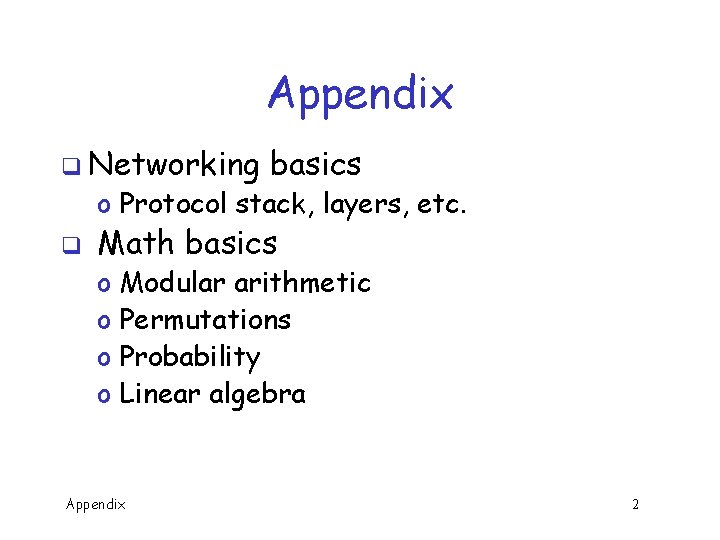Appendix q Networking basics o Protocol stack, layers, etc. q Math basics o o Modular arithmetic Permutations Probability Linear algebra Appendix 2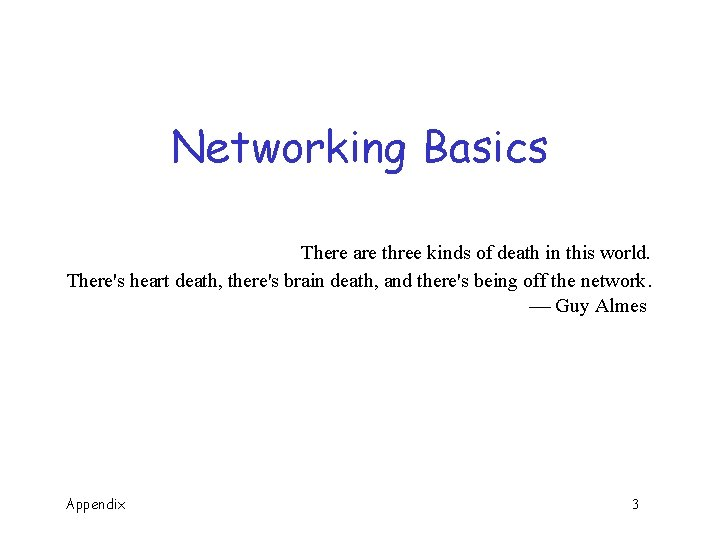Networking Basics There are three kinds of death in this world. There's heart death, there's brain death, and there's being off the network. Guy Almes Appendix 3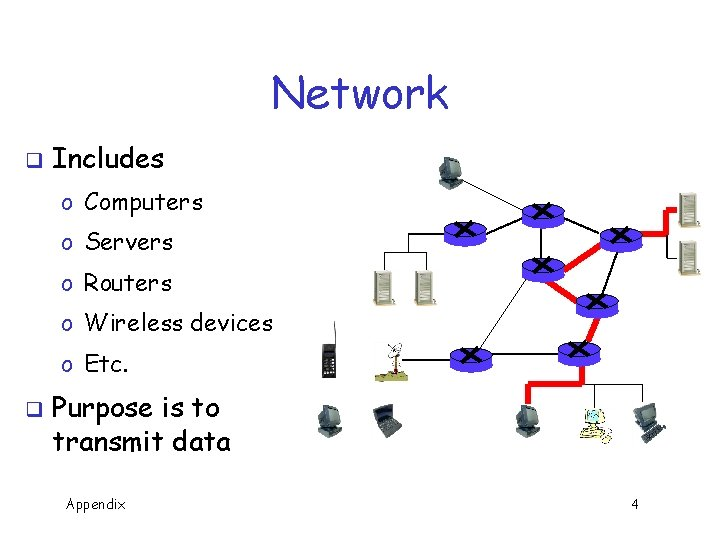Network q Includes o Computers o Servers o Routers o Wireless devices o Etc. q Purpose is to transmit data Appendix 4Network Edge q q Network edge includes… …Hosts o Computers o Laptops o Servers o Cell phones o Etc. , etc. Appendix 5Network Core q Network core consists of o Interconnected mesh of routers q Purpose is to move data from host to host Appendix 6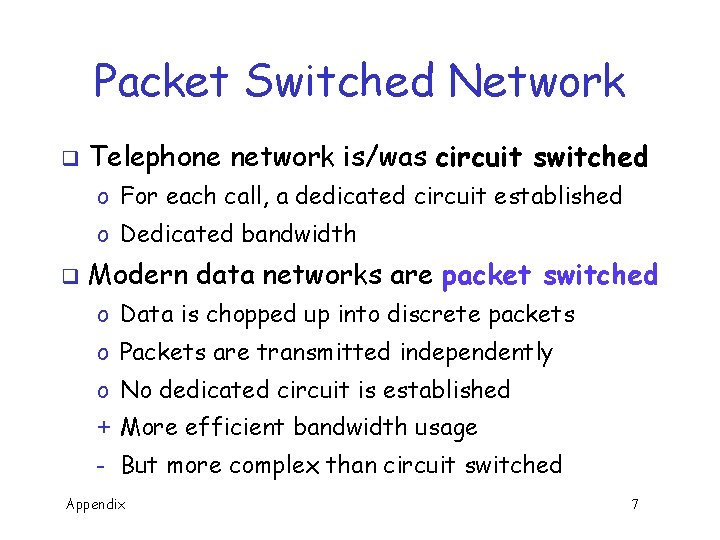Packet Switched Network q Telephone network is/was circuit switched o For each call, a dedicated circuit established o Dedicated bandwidth q Modern data networks are packet switched o Data is chopped up into discrete packets o Packets are transmitted independently o No dedicated circuit is established + More efficient bandwidth usage - But more complex than circuit switched Appendix 7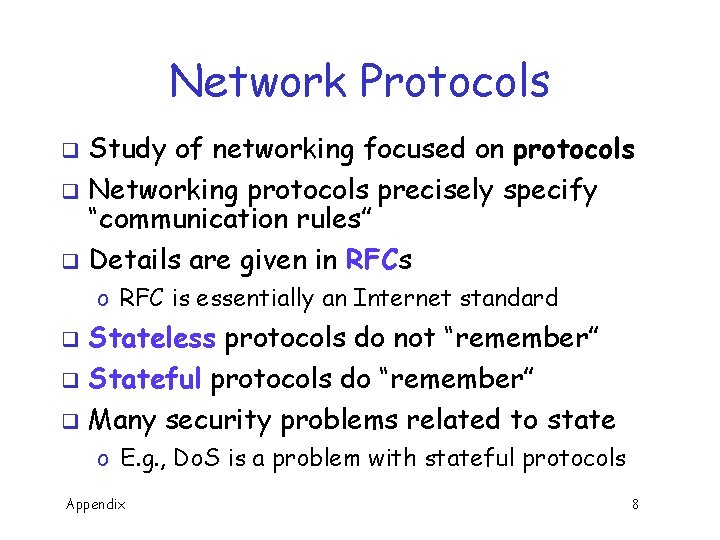Network Protocols Study of networking focused on protocols q Networking protocols precisely specify “communication rules” q Details are given in RFCs q o RFC is essentially an Internet standard Stateless protocols do not “remember” q Stateful protocols do “remember” q Many security problems related to state q o E. g. , Do. S is a problem with stateful protocols Appendix 8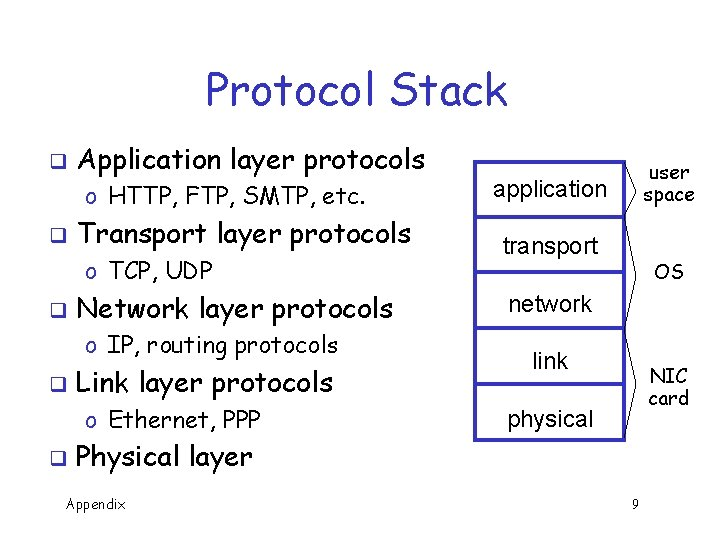Protocol Stack q Application layer protocols o HTTP, FTP, SMTP, etc. q Transport layer protocols o TCP, UDP q Network layer protocols o IP, routing protocols q Link layer protocols o Ethernet, PPP q user space application transport OS network link NIC card physical Physical layer Appendix 9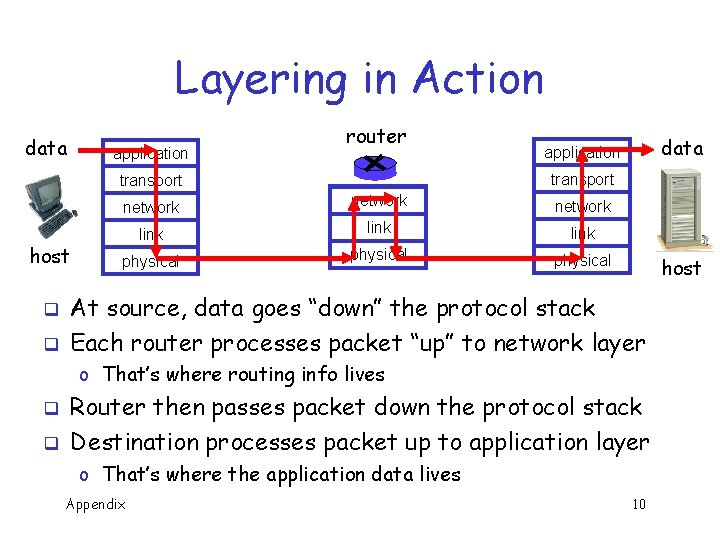Layering in Action data application router transport host q q data application network link physical host At source, data goes “down” the protocol stack Each router processes packet “up” to network layer o That’s where routing info lives q q Router then passes packet down the protocol stack Destination processes packet up to application layer o That’s where the application data lives Appendix 10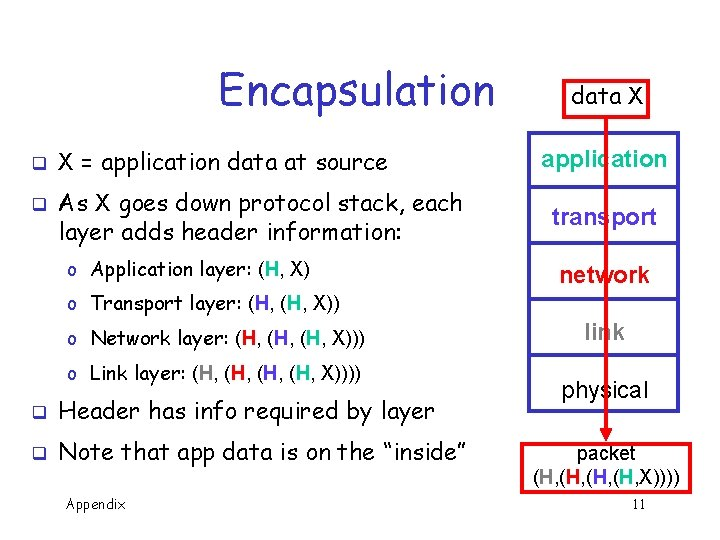Encapsulation q q X = application data at source As X goes down protocol stack, each layer adds header information: o Application layer: (H, X) data X application transport network o Transport layer: (H, X)) o Network layer: (H, (H, X))) o Link layer: (H, (H, X)))) q Header has info required by layer q Note that app data is on the “inside” Appendix link physical packet (H, (H, X)))) 11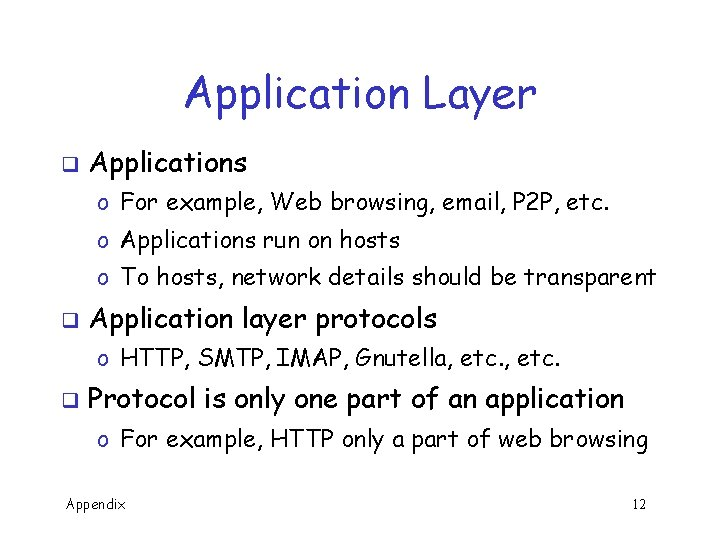Application Layer q Applications o For example, Web browsing, email, P 2 P, etc. o Applications run on hosts o To hosts, network details should be transparent q Application layer protocols o HTTP, SMTP, IMAP, Gnutella, etc. q Protocol is only one part of an application o For example, HTTP only a part of web browsing Appendix 12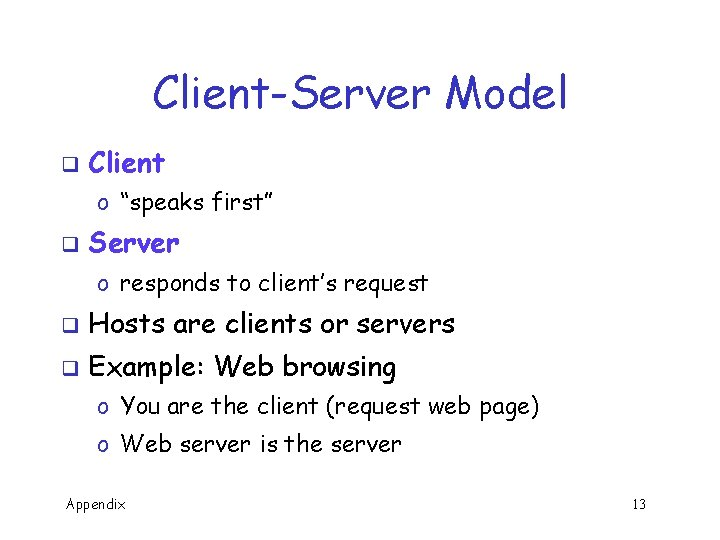Client-Server Model q Client o “speaks first” q Server o responds to client’s request q Hosts are clients or servers q Example: Web browsing o You are the client (request web page) o Web server is the server Appendix 13Peer-to-Peer Paradigm q Hosts q For act as clients and servers example, when sharing music o You are client when requesting a file o You are a server when someone downloads a file from you q In P 2 P, how does client find server? o Many different P 2 P models for this Appendix 14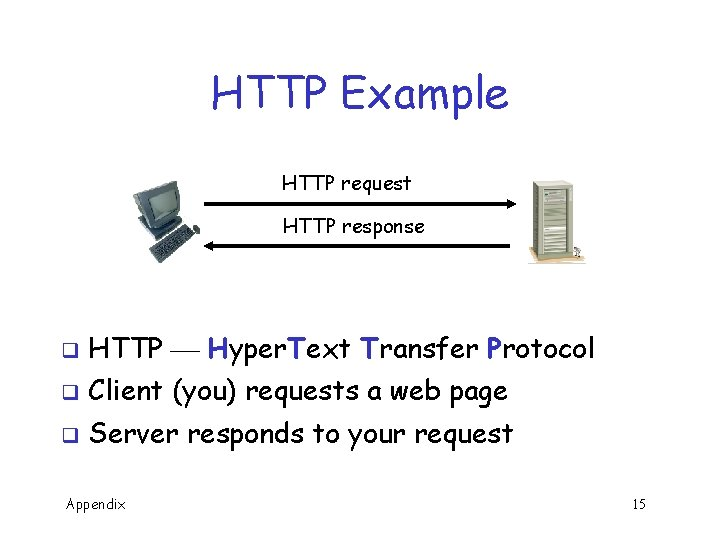HTTP Example HTTP request HTTP response q HTTP Hyper. Text Transfer Protocol q Client (you) requests a web page q Server responds to your request Appendix 15initial session cookie HTTP cookie later session Web Cookies reques respon t se, coo kie o c , t s e qu HTTP re onse p s e r P HTT Cookie database q HTTP is stateless cookies used to add state q Initially, cookie sent from server to browser q Browser manages cookie, sends it to server q Server uses cookie database to “remember” you Appendix 16Web Cookies q Web cookies used for… o Shopping carts, recommendations, etc. o A very (very) weak form of authentication q Privacy concerns o Web site can learn a lot about you o Multiple web sites could learn even more Appendix 17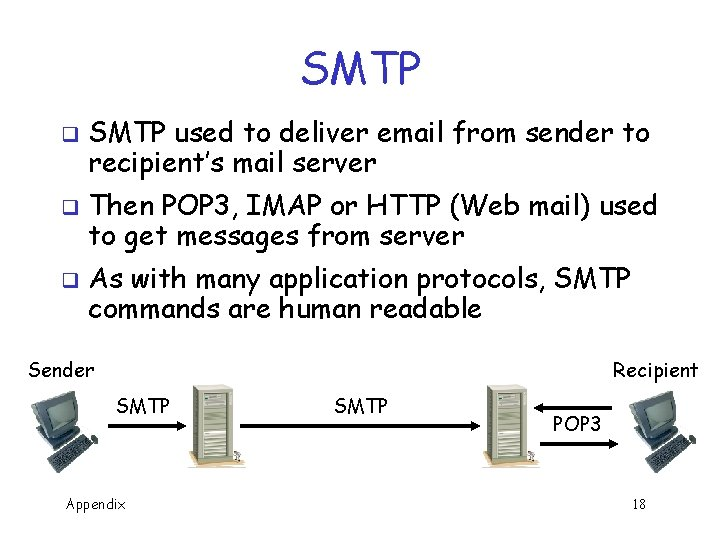SMTP q q q SMTP used to deliver email from sender to recipient’s mail server Then POP 3, IMAP or HTTP (Web mail) used to get messages from server As with many application protocols, SMTP commands are human readable Recipient Sender SMTP Appendix SMTP POP 3 18Spoofed email with SMTP User types the red lines: > telnet eniac. cs. sjsu. edu 25 220 eniac. sjsu. edu HELO ca. gov 250 Hello ca. gov, pleased to meet you MAIL FROM: <[email protected] gov> 250 [email protected] gov. . . Sender ok RCPT TO: <[email protected] sjsu. edu> 250 [email protected] sjsu. edu. . . Recipient ok DATA 354 Enter mail, end with ". " on a line by itself It is my pleasure to inform you that you are terminated. 250 Message accepted for delivery QUIT 221 eniac. sjsu. edu closing connection Appendix 19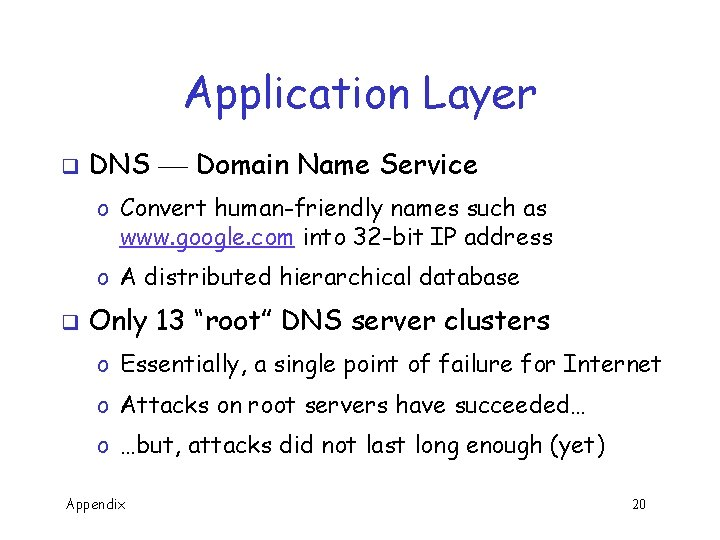Application Layer q DNS Domain Name Service o Convert human-friendly names such as www. google. com into 32 -bit IP address o A distributed hierarchical database q Only 13 “root” DNS server clusters o Essentially, a single point of failure for Internet o Attacks on root servers have succeeded… o …but, attacks did not last long enough (yet) Appendix 20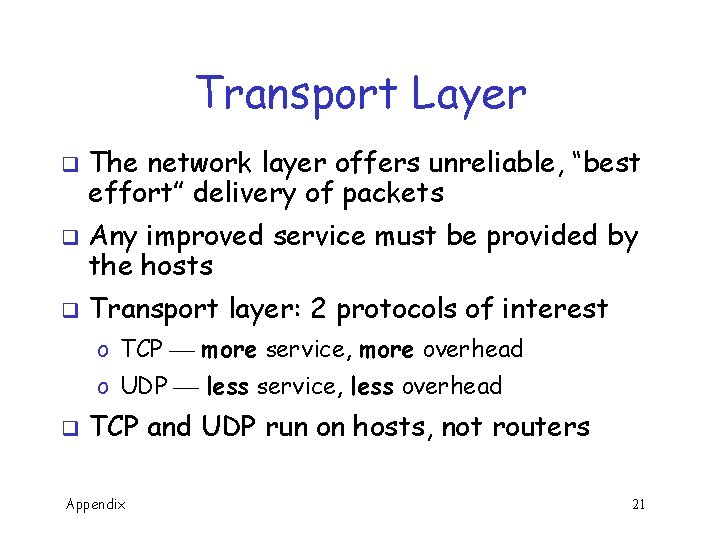Transport Layer q q q The network layer offers unreliable, “best effort” delivery of packets Any improved service must be provided by the hosts Transport layer: 2 protocols of interest o TCP more service, more overhead o UDP less service, less overhead q TCP and UDP run on hosts, not routers Appendix 21TCP q TCP assures that packets… o Arrive at destination o Are processed in order o Are not sent too fast for receiver: flow control q TCP also attempts to provide… o Network-wide congestion control q TCP is connection-oriented o TCP contacts server before sending data o Orderly setup and take down of “connection” o But no true connection, only logical “connection” Appendix 22TCP Header 0 8 bits 16 24 31 Source Port Offset Destination Port Sequence Number Acknowledgement Number reserved U A P R S F Window Checksum Urgent Pointer Options Padding Data (variable length) Source and destination port q Sequence number q Flags (ACK, SYN, RST, etc. ) q Header usually 20 bytes (if no options) q Appendix 23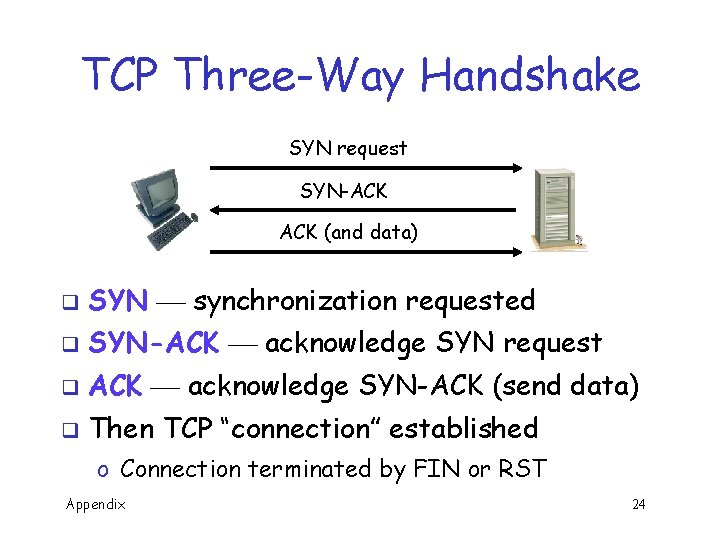TCP Three-Way Handshake SYN request SYN-ACK (and data) q SYN synchronization requested q SYN-ACK acknowledge SYN request q ACK acknowledge SYN-ACK (send data) q Then TCP “connection” established o Connection terminated by FIN or RST Appendix 24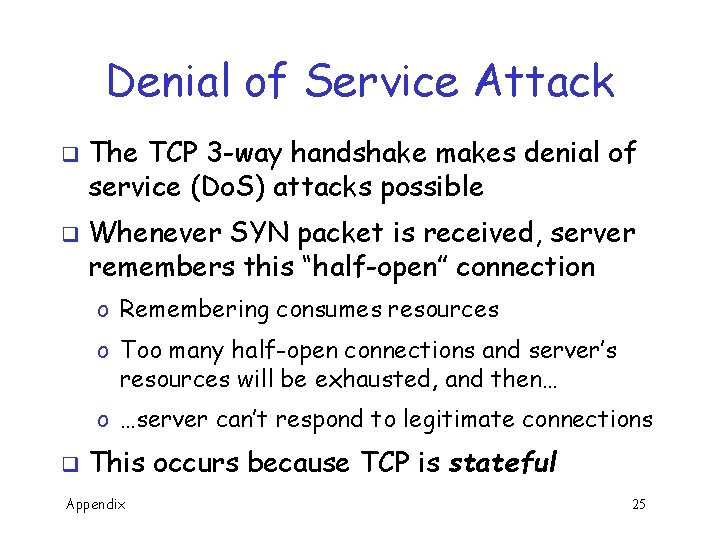Denial of Service Attack q q The TCP 3 -way handshake makes denial of service (Do. S) attacks possible Whenever SYN packet is received, server remembers this “half-open” connection o Remembering consumes resources o Too many half-open connections and server’s resources will be exhausted, and then… o …server can’t respond to legitimate connections q This occurs because TCP is stateful Appendix 25UDP q UDP is minimalist, “no frills” service o No assurance that packets arrive o No assurance packets are in order, etc. q Why does UDP exist? o More efficient (header only 8 bytes) o No flow control to slow down sender o No congestion control to slow down sender q If packets sent too fast, will be dropped o Either at intermediate router or at destination o But in some apps this may be OK (audio/video) Appendix 26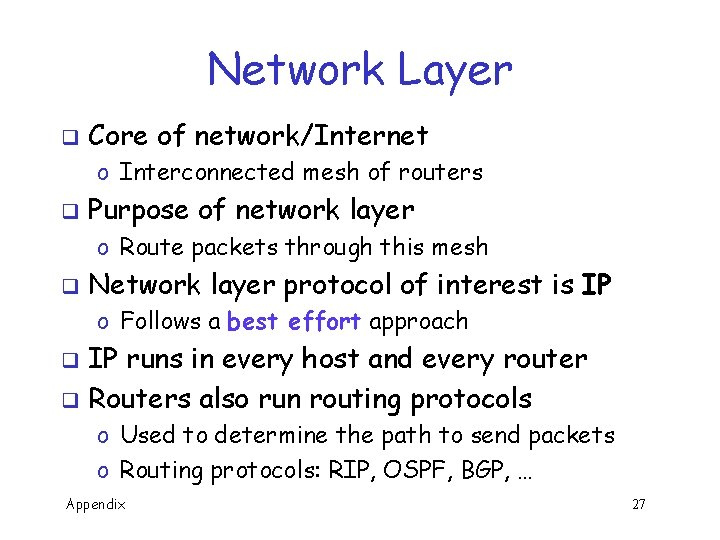Network Layer q Core of network/Internet o Interconnected mesh of routers q Purpose of network layer o Route packets through this mesh q Network layer protocol of interest is IP o Follows a best effort approach IP runs in every host and every router q Routers also run routing protocols q o Used to determine the path to send packets o Routing protocols: RIP, OSPF, BGP, … Appendix 27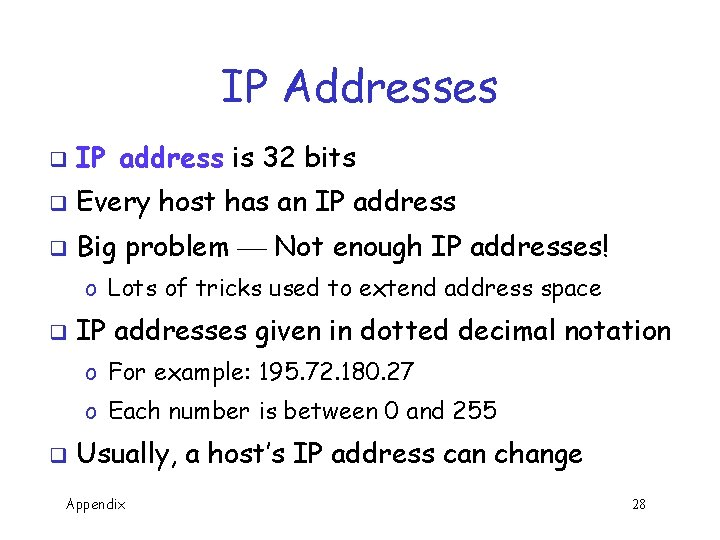IP Addresses q IP address is 32 bits q Every host has an IP address q Big problem Not enough IP addresses! o Lots of tricks used to extend address space q IP addresses given in dotted decimal notation o For example: 195. 72. 180. 27 o Each number is between 0 and 255 q Usually, a host’s IP address can change Appendix 28Socket Each host has a 32 bit IP address q But, many processes can run on one host q o E. g. , you can browse web, send email at same time How to distinguish processes on a host? q Each process has a 16 bit port number q o Numbers below 1024 are “well-known” ports (HTTP is port 80, POP 3 is port 110, etc. ) o Port numbers above 1024 are dynamic (as needed) q IP address + port number = socket o Socket uniquely identifies process, Internet-wide Appendix 29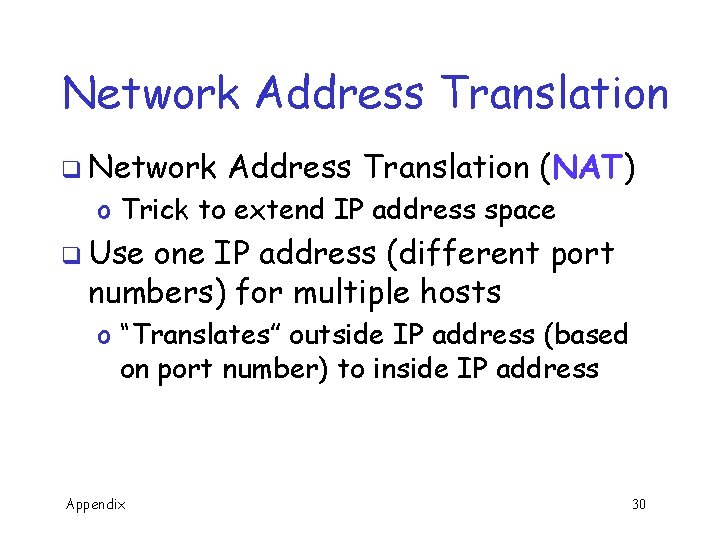Network Address Translation q Network Address Translation (NAT) o Trick to extend IP address space q Use one IP address (different port numbers) for multiple hosts o “Translates” outside IP address (based on port number) to inside IP address Appendix 30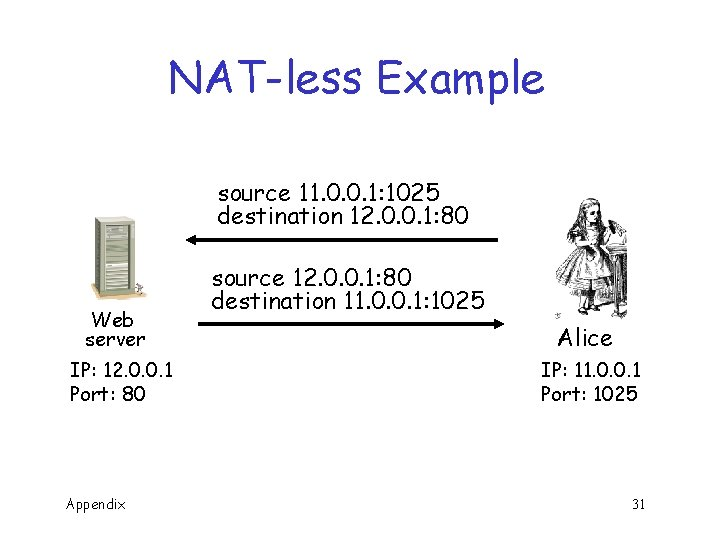NAT-less Example source 11. 0. 0. 1: 1025 destination 12. 0. 0. 1: 80 Web server IP: 12. 0. 0. 1 Port: 80 Appendix source 12. 0. 0. 1: 80 destination 11. 0. 0. 1: 1025 Alice IP: 11. 0. 0. 1 Port: 1025 31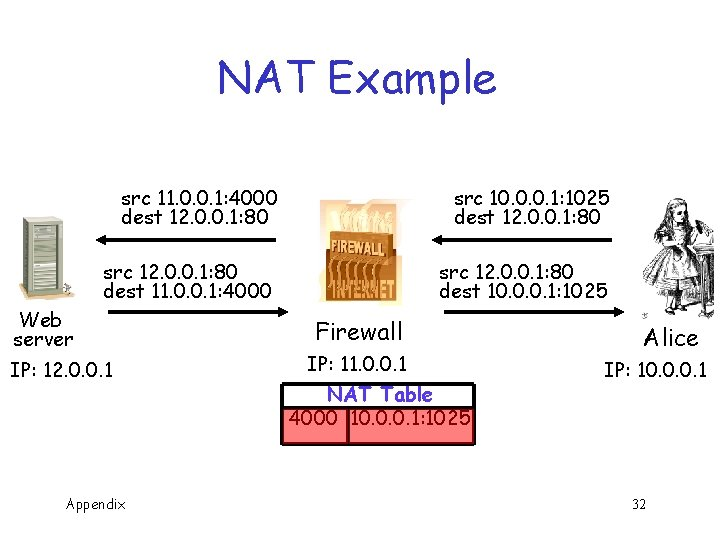NAT Example src 11. 0. 0. 1: 4000 dest 12. 0. 0. 1: 80 src 10. 0. 0. 1: 1025 dest 12. 0. 0. 1: 80 src 12. 0. 0. 1: 80 dest 11. 0. 0. 1: 4000 src 12. 0. 0. 1: 80 dest 10. 0. 0. 1: 1025 Web server IP: 12. 0. 0. 1 Appendix Firewall IP: 11. 0. 0. 1 NAT Table 4000 10. 0. 0. 1: 1025 Alice IP: 10. 0. 0. 1 32NAT: The Last Word q Advantage(s)? o Extends IP address space o One (or a few) IP address(es) can be shared by many users q Disadvantage(s)? o End-to-end security is more difficult o Might make IPSec less effective (IPSec discussed in Chapter 10) Appendix 33IP Header q IP header has necessary info for routers q Time to live (TTL) limits number of “hops” q Fragmentation information (see next slide) o E. g. , source and destination IP addresses o So packets can’t circulate forever Appendix 34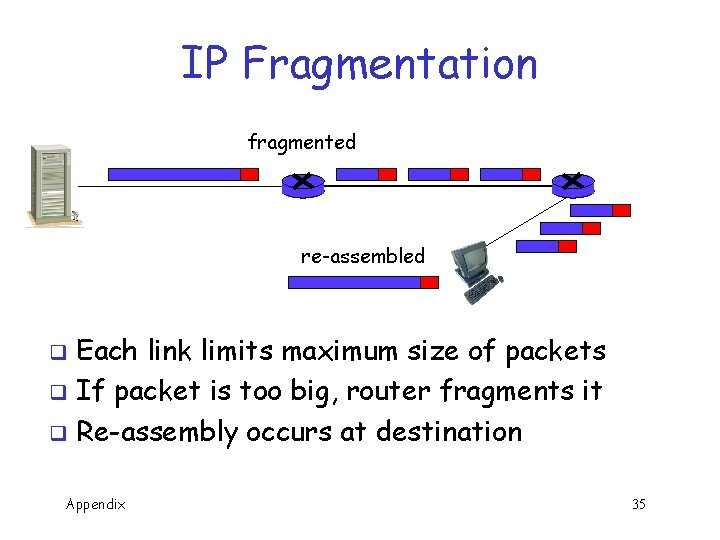IP Fragmentation fragmented re-assembled Each link limits maximum size of packets q If packet is too big, router fragments it q Re-assembly occurs at destination q Appendix 35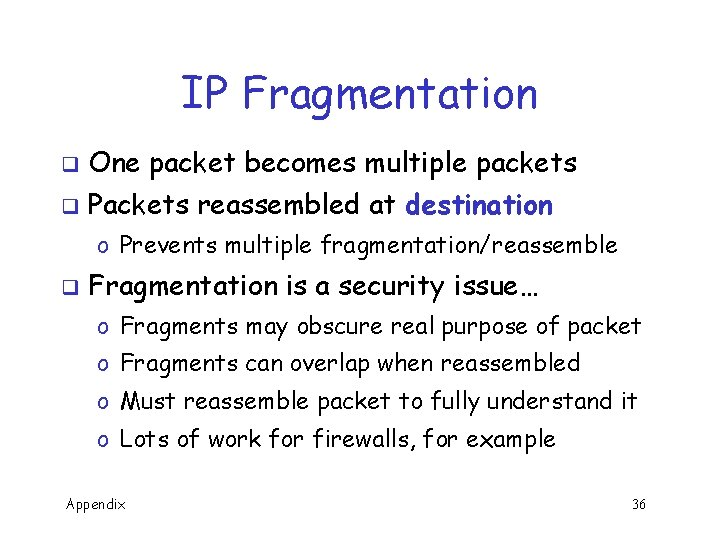IP Fragmentation q One packet becomes multiple packets q Packets reassembled at destination o Prevents multiple fragmentation/reassemble q Fragmentation is a security issue… o Fragments may obscure real purpose of packet o Fragments can overlap when reassembled o Must reassemble packet to fully understand it o Lots of work for firewalls, for example Appendix 36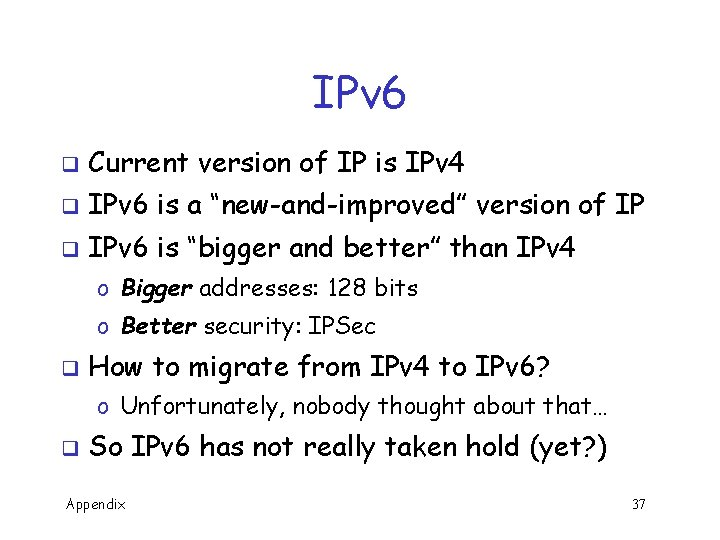IPv 6 q Current version of IP is IPv 4 q IPv 6 is a “new-and-improved” version of IP q IPv 6 is “bigger and better” than IPv 4 o Bigger addresses: 128 bits o Better security: IPSec q How to migrate from IPv 4 to IPv 6? o Unfortunately, nobody thought about that… q So IPv 6 has not really taken hold (yet? ) Appendix 37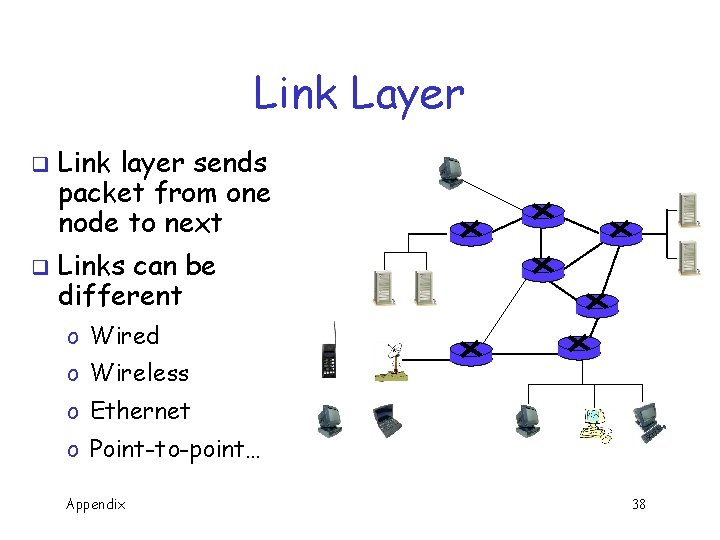Link Layer q q Link layer sends packet from one node to next Links can be different o Wired o Wireless o Ethernet o Point-to-point… Appendix 38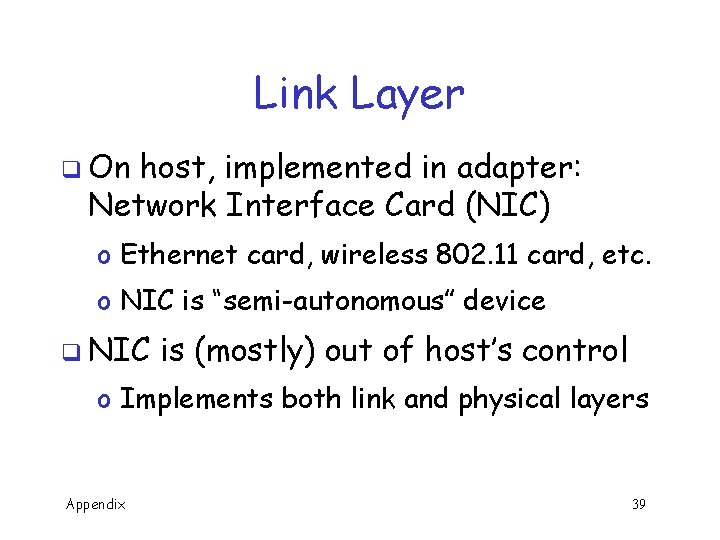Link Layer q On host, implemented in adapter: Network Interface Card (NIC) o Ethernet card, wireless 802. 11 card, etc. o NIC is “semi-autonomous” device q NIC is (mostly) out of host’s control o Implements both link and physical layers Appendix 39Ethernet q Ethernet is a multiple access protocol q Many hosts access a shared media o On a local area network, or LAN q With multiple access, packets can “collide” o Data is corrupted and packets must be resent q How to efficiently deal with collisions in distributed environment? o Many possibilities, ethernet is most popular q We won’t discuss details here… Appendix 40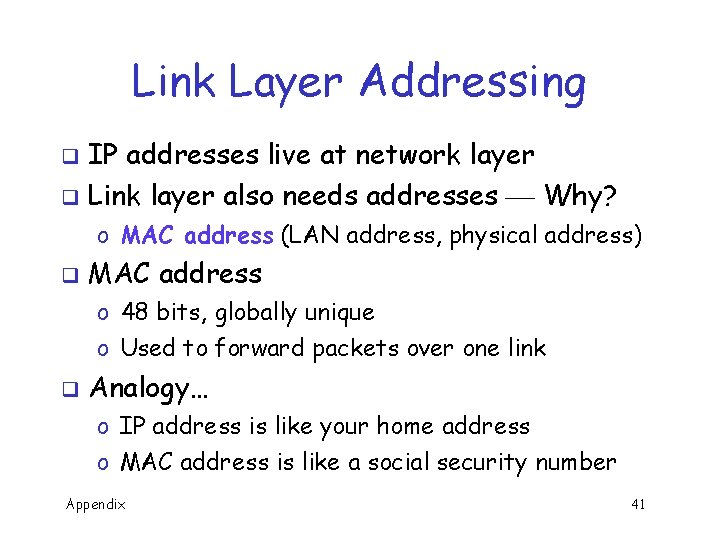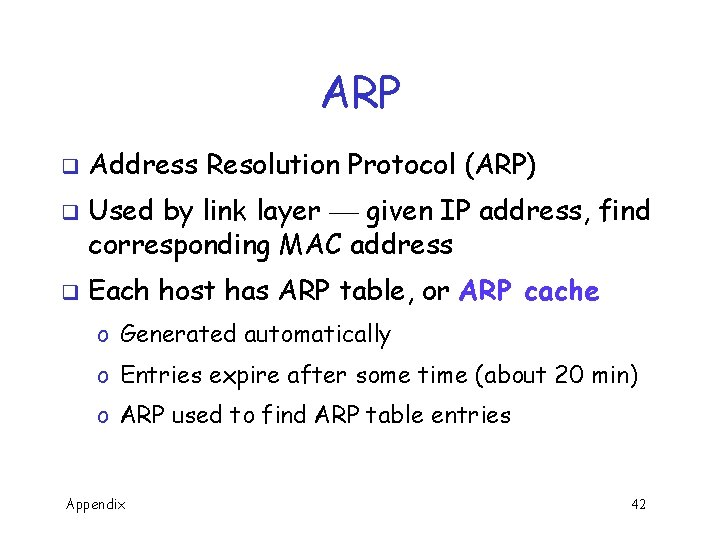ARP q q q Address Resolution Protocol (ARP) Used by link layer given IP address, find corresponding MAC address Each host has ARP table, or ARP cache o Generated automatically o Entries expire after some time (about 20 min) o ARP used to find ARP table entries Appendix 42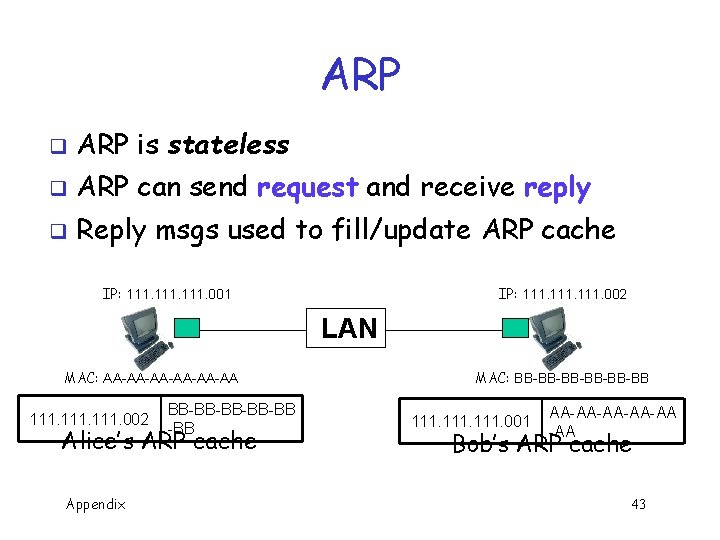ARP q ARP is stateless q ARP can send request and receive reply q Reply msgs used to fill/update ARP cache IP: 111. 001 IP: 111. 002 LAN MAC: AA-AA-AA-AA 111. 002 BB-BB-BB -BB Alice’s ARP cache Appendix MAC: BB-BB-BB-BB 111. 001 AA-AA-AA -AA Bob’s ARP cache 43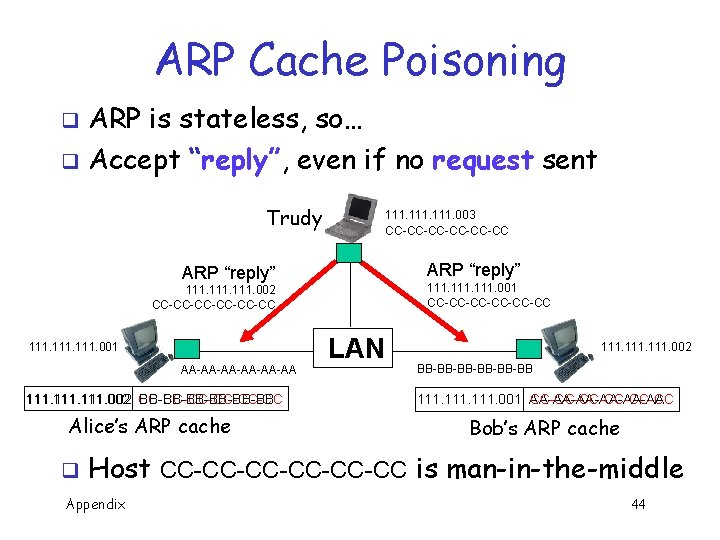ARP Cache Poisoning ARP is stateless, so… q Accept “reply”, even if no request sent q Trudy 111. 003 CC-CC-CC-CC ARP “reply” 111. 001 CC-CC-CC-CC 111. 002 CC-CC-CC-CC 111. 001 AA-AA-AA-AA 111. 002 CC-CC-CC-CC BB-BB-BB-BB Alice’s ARP cache q LAN 111. 002 BB-BB-BB-BB 111. 001 AA-AA-AA-AA CC-CC-CC-CC Bob’s ARP cache Host CC-CC-CC-CC is man-in-the-middle Appendix 44Math Basics 7/5 ths of all people don’t understand fractions. Anonymous Appendix 45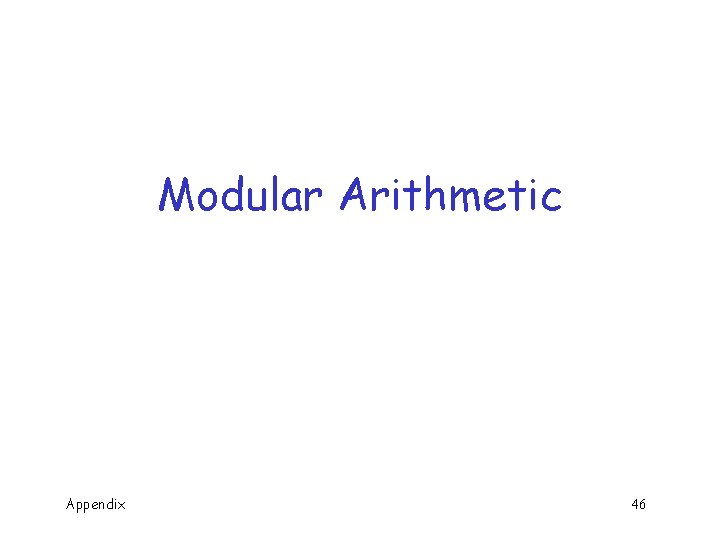Modular Arithmetic Appendix 46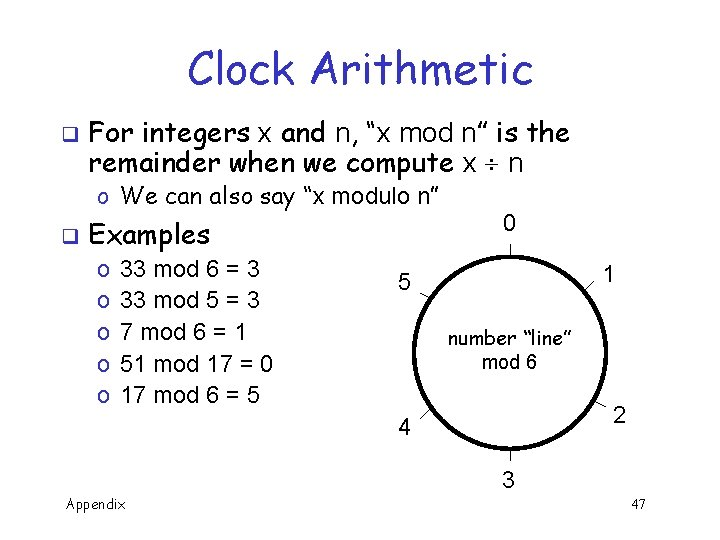Clock Arithmetic q For integers x and n, “x mod n” is the remainder when we compute x n o We can also say “x modulo n” q Examples o o o 33 mod 6 = 3 33 mod 5 = 3 7 mod 6 = 1 51 mod 17 = 0 17 mod 6 = 5 0 1 5 number “line” mod 6 2 4 3 Appendix 47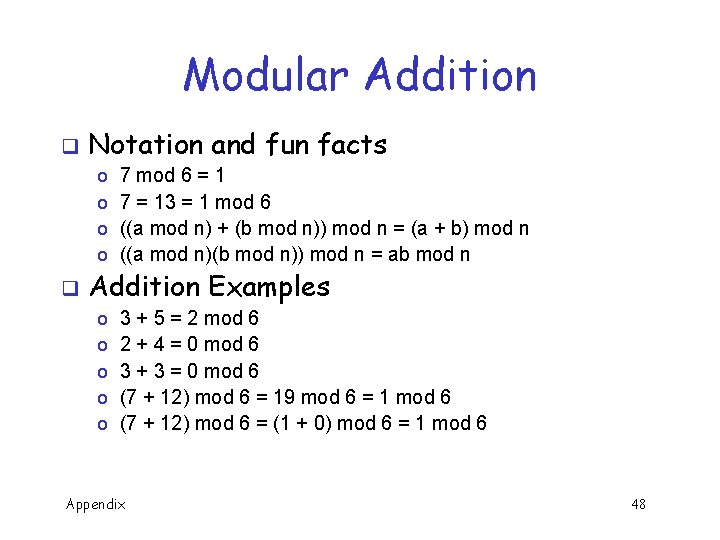Modular Addition q q Notation and fun facts o o 7 mod 6 = 1 7 = 13 = 1 mod 6 ((a mod n) + (b mod n)) mod n = (a + b) mod n ((a mod n)(b mod n)) mod n = ab mod n o o o 3 + 5 = 2 mod 6 2 + 4 = 0 mod 6 3 + 3 = 0 mod 6 (7 + 12) mod 6 = 19 mod 6 = 1 mod 6 (7 + 12) mod 6 = (1 + 0) mod 6 = 1 mod 6 Addition Examples Appendix 48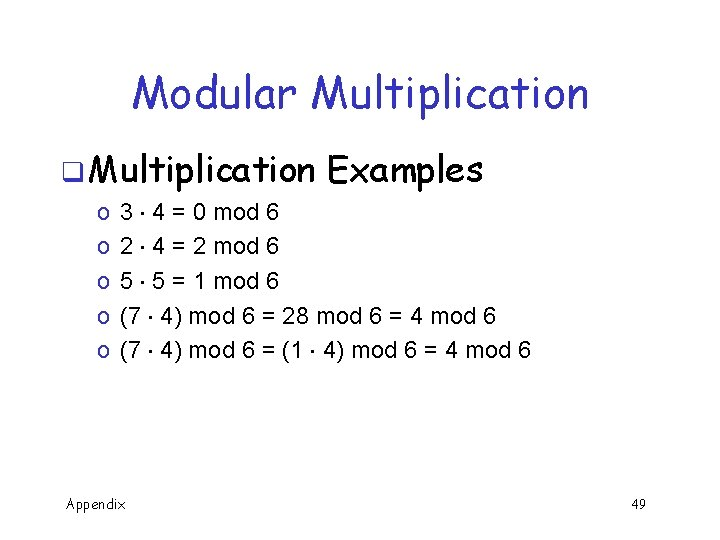Modular Multiplication q Multiplication o o o Examples 3 4 = 0 mod 6 2 4 = 2 mod 6 5 5 = 1 mod 6 (7 4) mod 6 = 28 mod 6 = 4 mod 6 (7 4) mod 6 = (1 4) mod 6 = 4 mod 6 Appendix 49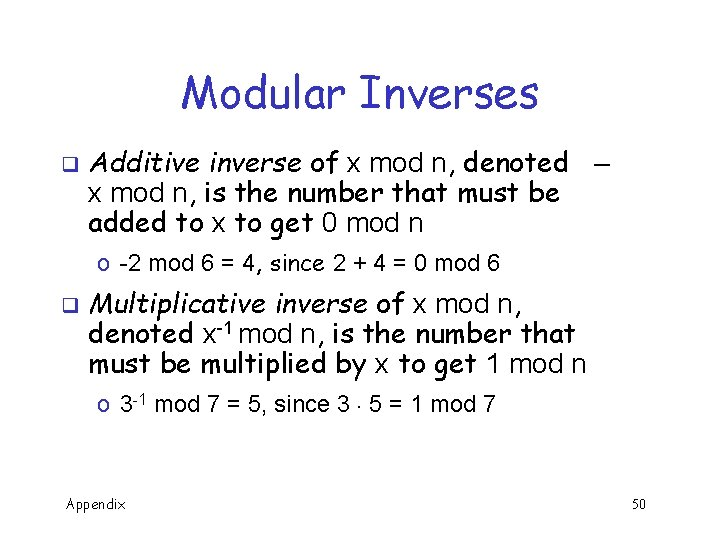Modular Inverses q Additive inverse of x mod n, denoted – x mod n, is the number that must be added to x to get 0 mod n o -2 mod 6 = 4, since 2 + 4 = 0 mod 6 q Multiplicative inverse of x mod n, denoted x-1 mod n, is the number that must be multiplied by x to get 1 mod n o 3 -1 mod 7 = 5, since 3 5 = 1 mod 7 Appendix 50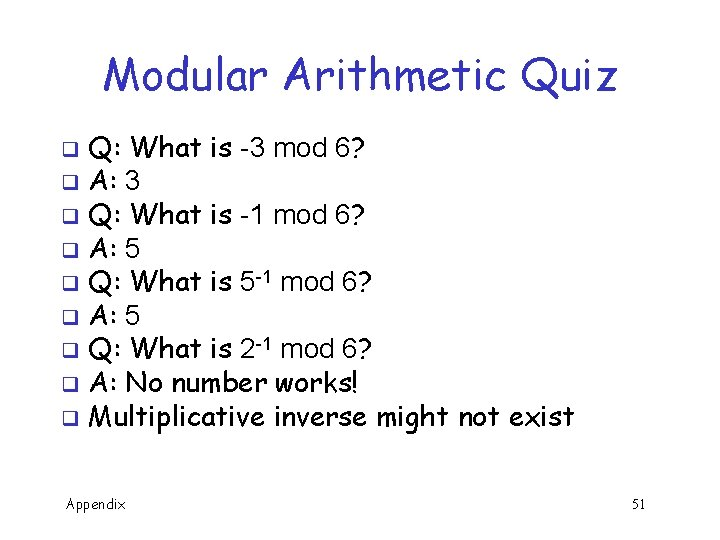Modular Arithmetic Quiz Q: What is -3 mod 6? q A: 3 q Q: What is -1 mod 6? q A: 5 q Q: What is 5 -1 mod 6? q A: 5 q Q: What is 2 -1 mod 6? q A: No number works! q Multiplicative inverse might not exist q Appendix 51Relative Primality and y are relatively prime if they have no common factor other than 1 q x-1 mod y exists only when x and y are relatively prime q If it exists, x-1 mod y is easy to compute using Euclidean Algorithm qx o We won’t do the computation here o But, an efficient algorithm exists Appendix 52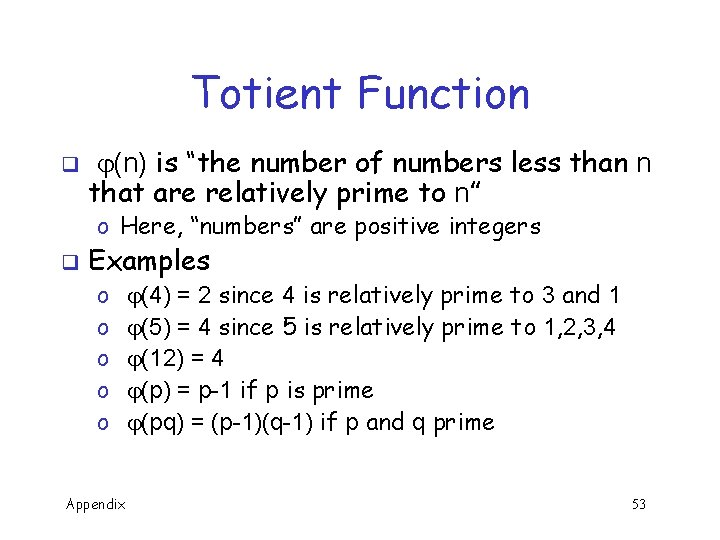Totient Function q (n) is “the number of numbers less than n that are relatively prime to n” o Here, “numbers” are positive integers q Examples o o o Appendix (4) = 2 since 4 is relatively prime to 3 and 1 (5) = 4 since 5 is relatively prime to 1, 2, 3, 4 (12) = 4 (p) = p-1 if p is prime (pq) = (p-1)(q-1) if p and q prime 53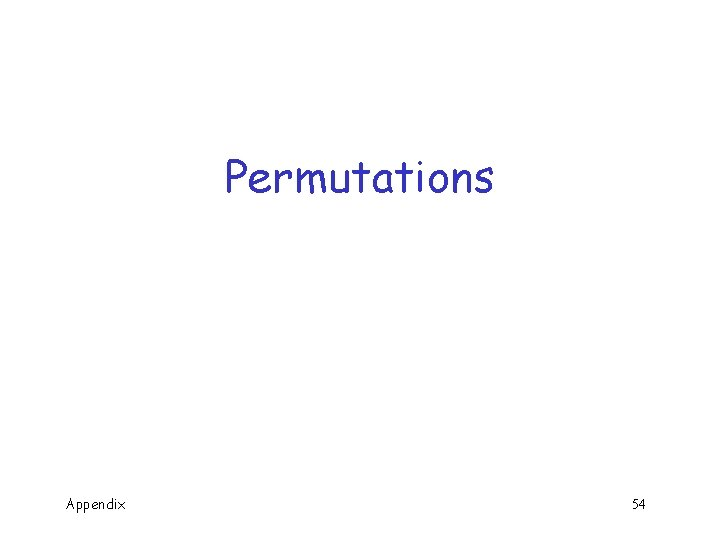Permutations Appendix 54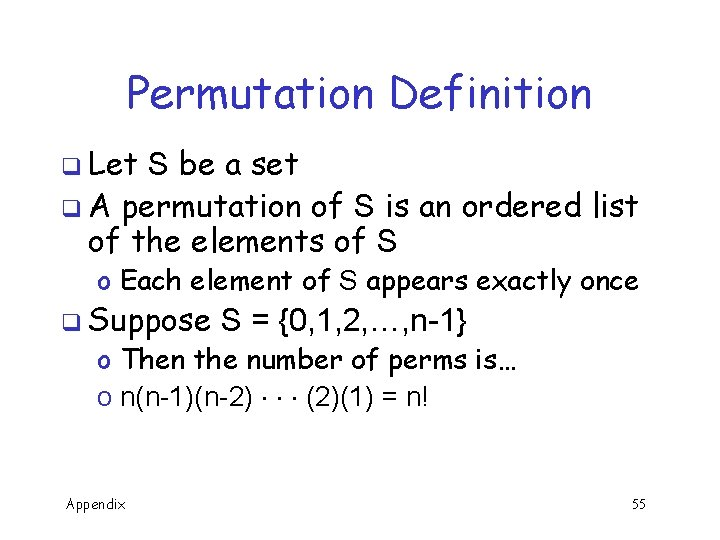Permutation Definition q Let S be a set q A permutation of S is an ordered list of the elements of S o Each element of S appears exactly once q Suppose S = {0, 1, 2, …, n-1} o Then the number of perms is… o n(n-1)(n-2) (2)(1) = n! Appendix 55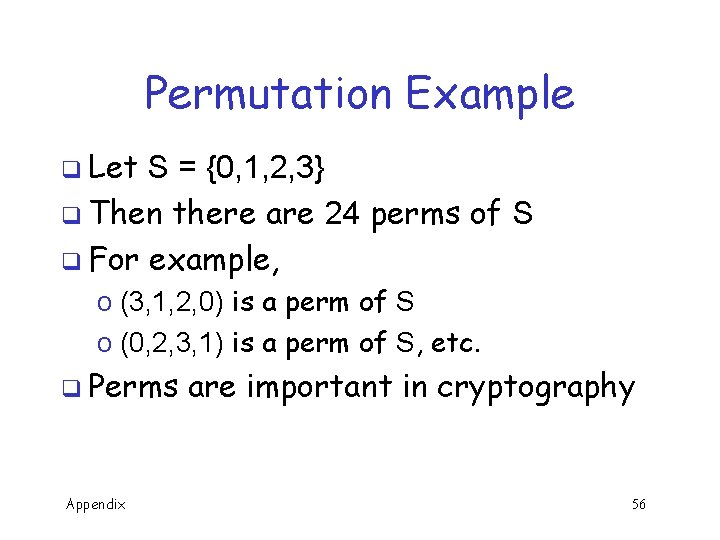Permutation Example q Let S = {0, 1, 2, 3} q Then there are 24 perms of S q For example, o (3, 1, 2, 0) is a perm of S o (0, 2, 3, 1) is a perm of S, etc. q Perms Appendix are important in cryptography 56Probability Basics Appendix 57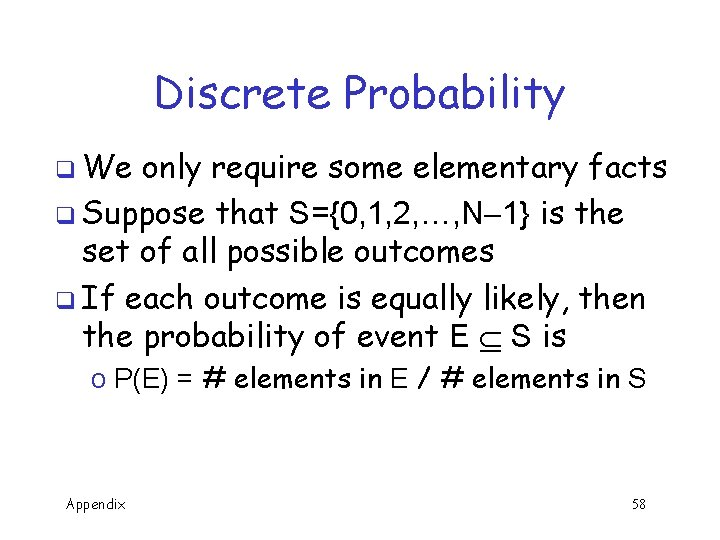Discrete Probability q We only require some elementary facts q Suppose that S={0, 1, 2, …, N 1} is the set of all possible outcomes q If each outcome is equally likely, then the probability of event E S is o P(E) = # elements in E / # elements in S Appendix 58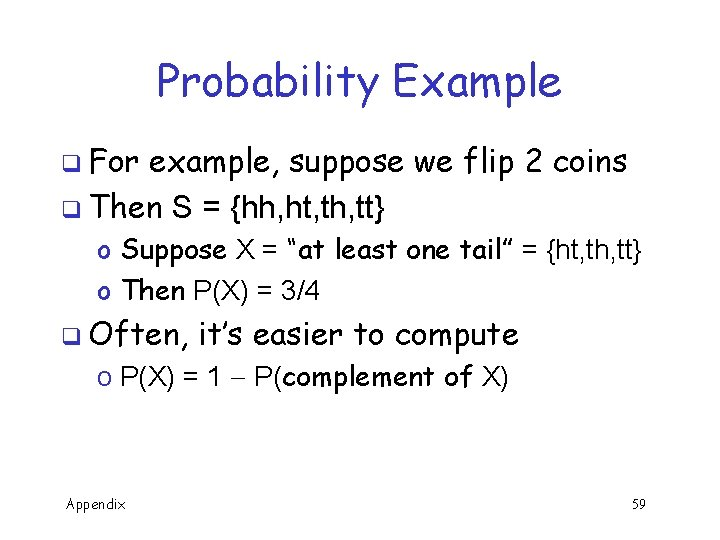Probability Example q For example, suppose we flip 2 coins q Then S = {hh, ht, th, tt} o Suppose X = “at least one tail” = {ht, th, tt} o Then P(X) = 3/4 q Often, it’s easier to compute o P(X) = 1 P(complement of X) Appendix 59Complement q Again, suppose we flip 2 coins q Let S = {hh, ht, th, tt} o Suppose X = “at least one tail” = {ht, th, tt} o Complement of X is “no tails” = {hh} q Then o P(X) = 1 P(comp. of X) = 1 1/4 = 3/4 q We Appendix make use of this trick often! 60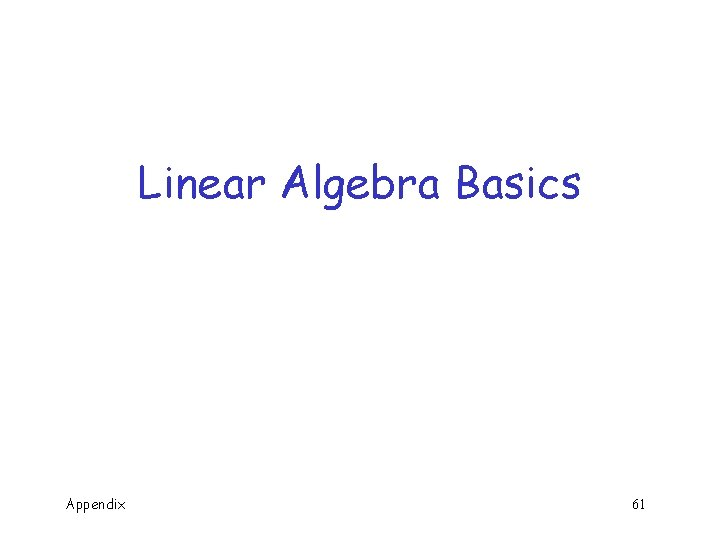Linear Algebra Basics Appendix 61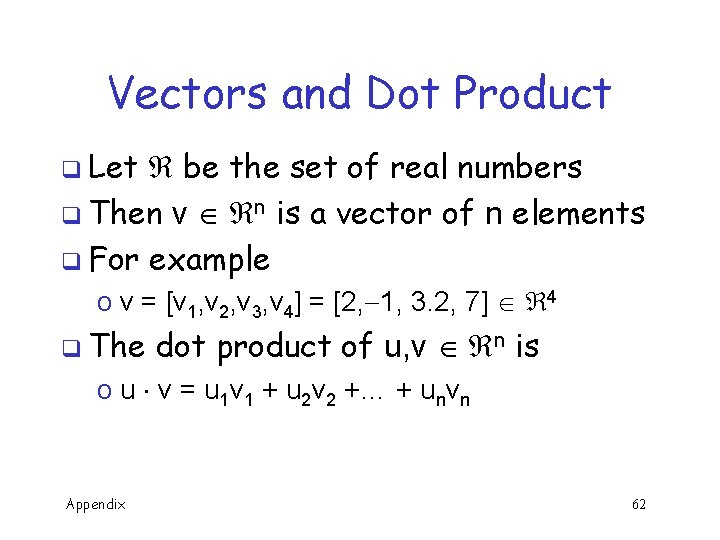Vectors and Dot Product q Let be the set of real numbers q Then v n is a vector of n elements q For example o v = [v 1, v 2, v 3, v 4] = [2, 1, 3. 2, 7] 4 q The dot product of u, v n is o u v = u 1 v 1 + u 2 v 2 +… + unvn Appendix 62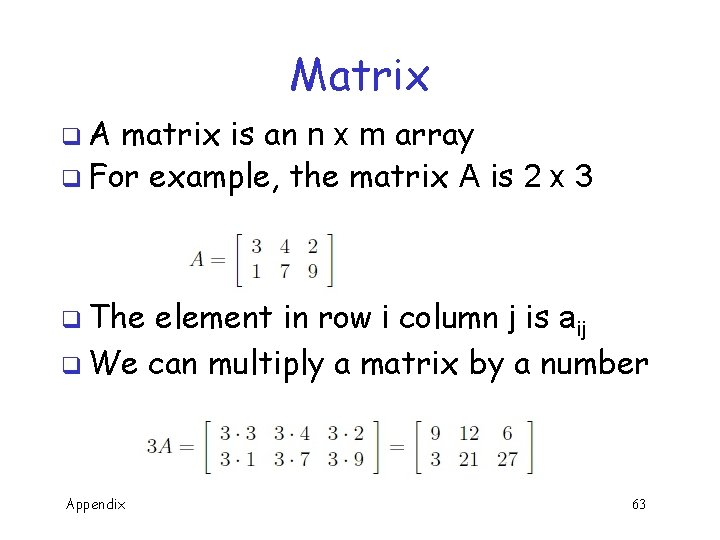Matrix q. A matrix is an n x m array q For example, the matrix A is 2 x 3 q The element in row i column j is aij q We can multiply a matrix by a number Appendix 63Matrix Addition q We can add matrices of the same size q We can also multiply matrices, but this is not so obvious q We do not simply multiply the elements Appendix 64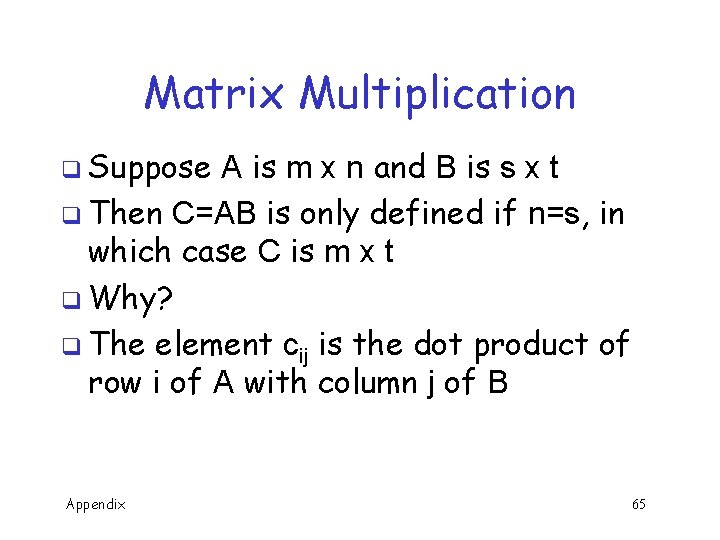Matrix Multiplication q Suppose A is m x n and B is s x t q Then C=AB is only defined if n=s, in which case C is m x t q Why? q The element cij is the dot product of row i of A with column j of B Appendix 65Matrix Multiply Example q Suppose q Then q And Appendix AB is undefined 66Matrix Multiply Useful Fact Consider AU = B where A is a matrix and U and B are column vectors q Let a 1, a 2, …, an be columns of A and u 1, u 2, …, un the elements of U q Then B = u 1 a 1 + u 2 a 2 + … + unan q Example: [ 31 45] [ 26 ] Appendix = 2[ ] 3 1 + 6 [ ] 4 5 = [ 30 ] 32 67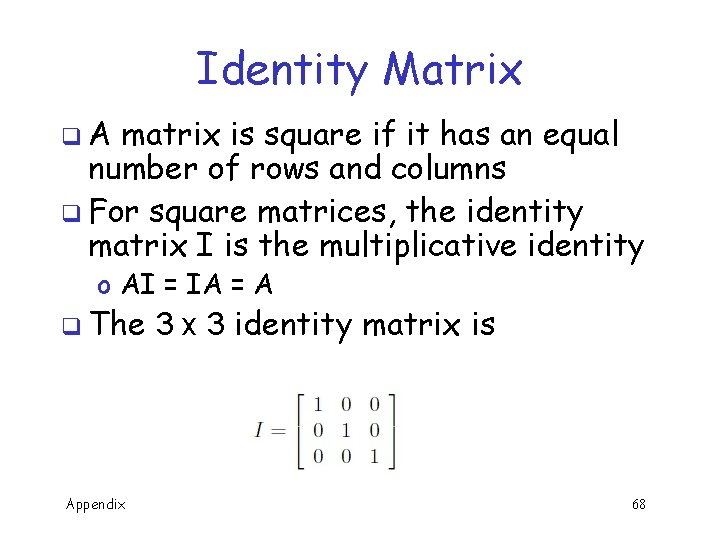Identity Matrix q. A matrix is square if it has an equal number of rows and columns q For square matrices, the identity matrix I is the multiplicative identity o AI = IA = A q The Appendix 3 identity matrix is 68Block Matricies Block matrices are matrices of matrices q For example q We can do arithmetic with block matrices q Block matrix multiplication works if individual matrix dimensions “match” q Appendix 69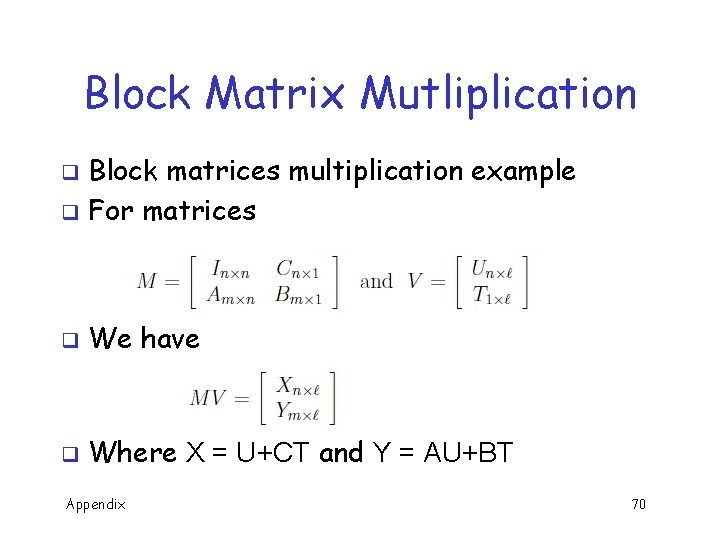Block Matrix Mutliplication Block matrices multiplication example q For matrices q q We have q Where X = U+CT and Y = AU+BT Appendix 70Linear Independence q Vectors u, v n linearly independent if au + bv = 0 implies a=b=0 q For example, q Are Appendix linearly independent 71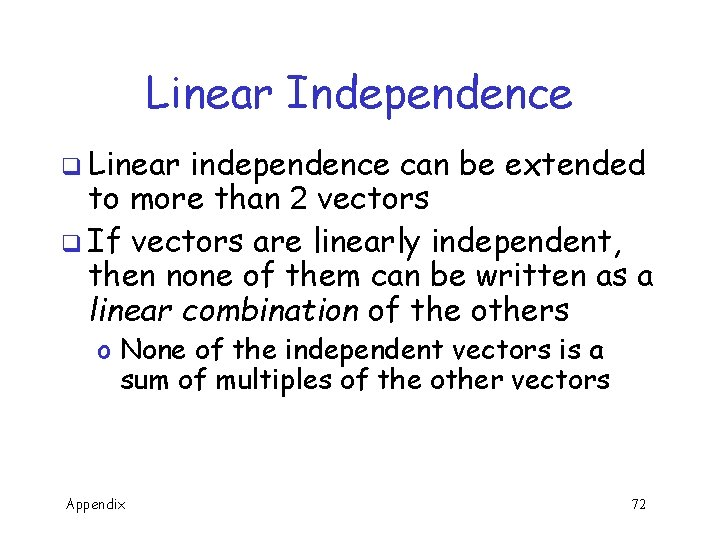Linear Independence q Linear independence can be extended to more than 2 vectors q If vectors are linearly independent, then none of them can be written as a linear combination of the others o None of the independent vectors is a sum of multiples of the other vectors Appendix 72